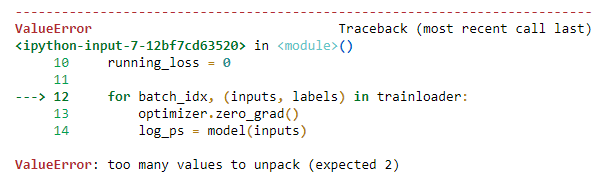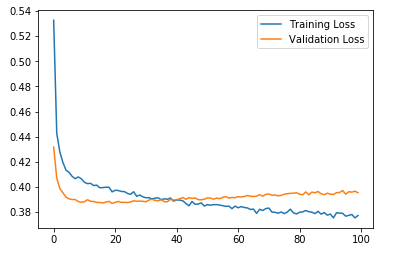# Problem with dimensions

I want to build a neural network classifier for binary class.
trainset.shape = (41712, 231)
testset.shape = (10429, 231)
It outputs:
ValueError Traceback (most recent call last)
in ()
10 running_loss = 0
11
—> 12 for inputs, labels in trainloader:
14 log_ps = model(inputs)

ValueError: too many values to unpack (expected 2)

This is the code:

``````transform = transforms.Compose([transforms.ToTensor(), transforms.Normalize((0.5, 0.5, 0.5), (0.5, 0.5, 0.5))])

class Classifier(nn.Module):
def __init__(self):
super().__init__()
self.fc1 = nn.Linear(230, 200)
self.fc2 = nn.Linear(200, 150)
self.fc3 = nn.Linear(150, 100)
self.fc4 = nn.Linear(100, 1)
def forward(self, x):
x = F.relu(self.fc1(x))
x = F.relu(self.fc2(x))
x = F.relu(self.fc3(x))
x = F.log_sigmoid(self.fc4(x), dim=1)
return x

model = Classifier()
criterion = nn.BCELoss()
train_losses, test_losses = [], []
for e in range(30):
running_loss = 0
log_ps = model(inputs)
loss = criterion(log_ps, labels)
loss.backward()
optimizer.step()
running_loss += loss.item()
``````

What is the problem here ?

the error is saying that your `trainloader` not returning two outputs, for this line to work correctly: `for inputs, labels in trainloader:`

Sorry I’m still not sure what should be modified flr this to work. Why doesn’t it return 2 outputs ?
I stacked my training data and their labels using this line of code to construct the trainloader.

``````trainset = np.column_stack([train_data.as_matrix(), train_labels.as_matrix()])
``````

Is that a wrong input? What is the assumed input ?

should like this in last for loop :
for batch_idx, (inputs, labels) in trainloader:

Still gives the same output.Try to create a `TensorDataset` passing the data and labels separately:

``````trainset = torch.utils.data.TensorDataset(
torch.from_numpy(train_data.as_matrix()),
torch.from_numpy(train_labels.as_matrix())
)
``````
1 Like

Thank you.
That solves the problem. The network trains but the loss is very high and the accuracy is very low compared to other models trained on the same data set. I think I have a problem with the loss function. If there is any hint or if there is a guide on how to choose the loss function, I would be grateful to have a look at it.
Kind Regards

`nn.BCELoss` expects a tensor containing probabilities, so you should replace the last `F.log_sigmoid` in your model with `F.sigmoid`.
Could you change it and try to fit your data again?

I changed it. The loss is now lower but still the accuracy is too low stuck at 9% compared to 83% with random forest on the same data.
I also tried changing the learning rate to see if it works but still it wiggles around the same accuracy.

By the way this is the code I use for calculating probability. I think it’s okay.

``````                ps = torch.exp(log_ps)
top_p, top_class = ps.topk(1, dim=1)
equals = top_class == labels.view(*top_class.shape)
accuracy = torch.mean(equals.type(torch.FloatTensor))
print(f'Accuracy: {accuracy.item()*100}%')
``````

I tried different loss functions. I tried changing the learning rate. I tried increasing and decreasing the batch size. But I still have the same problem. The accuracy is too low compared to other models that were used on the same data. It wiggles between 3% to 15% which pretty much random I guess.

You don’t need to use `torch.exp`, if you’ve used `F.sigmoid` in your model.
Also, you should use a threshold on the probabilities to get the corresponding class, e.g. `0.5`.
`tensor.topk` will return a zero tensor in your current setup.

1 Like

Thank you very much. That pretty much does it. The net trains and gives reasonable results.But the accuracy is wiggling vigorously, looks like a zigzag.I tried changing the learning rate and the batch size (now 1000) but it still outputs a zigzag. And calculating ROC_AUC is not very effective.

Do you have a class imbalance in your dataset?
Is the mean of the accuracy increasing for more training epochs are is it stays approx. at the same level?

Yes there is class imbalance 68:32. I tried under-sampling but it didn’t make much of a difference.
The mean accuracy increases to 84% by the 6th epoch, then bounces back and forth between 79% and 89%.
This happens with very big batch sizes. With small batch size it can even bounce between 75% and 97%.

Thanks for the info.
Are you plotting the accuracy for the whole epoch or for each batch?
Could you check the predictions of your model and see if they (mostly) belong to the majority class?

I’m plotting accuracy for the whole epoch.
Predictions are 73% belonging to majority, but the test labels are 69% belonging to majority. So it’s close to the true values.